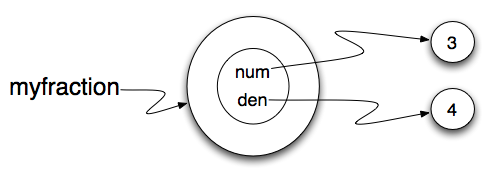# 18.1. Fractions¶

We have all had to work with fractions when we were younger. Or, perhaps you do a lot of cooking and have to manage measurements for ingredients. In any case, fractions are something that we are familiar with. In this chapter we will develop a class to represent a fraction including some of the most common methods that we would like fractions to be able to do.

A fraction is most commonly thought of as two integers, one over the other, with a line separating them. The number on the top is called the numerator and the number on the bottom is called the denominator. Sometimes people use a slash for the line and sometimes they use a straight line. The fact is that it really does not matter so long as you know which is the numerator and which is the denominator.

To design our class, we simply need to use the analysis above to realize that the state of a fraction object can be completely described by representing two integers. We can begin by implementing the Fraction class and the __init__ method which will allow the user to provide a numerator and a denominator for the fraction being created.

Note that the __str__ method provides a “typical” looking fraction using a slash between the numerator and denominator. The figure below shows the state of myfraction. We have also added a few simple accessor methods, getNum and getDen, that can return the state values for the fraction.